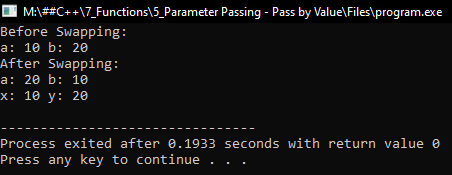# Parameter Pass by Value in C++

## Parameter Pass by Value in C++ with Examples

In this article, I am going to discuss Parameter Pass by Value in C++ Language with examples. Please read our previous article, where we discussed Default Arguments in C++ with examples.

##### Parameter Passing Techniques in C++

In this article, we will discuss parameter passing methods. We have already learned about functions, how to write the functions and how to call the functions. Now we will learn what are the methods, a function can take the parameter. So, a function can take parameters in 3 ways, that are,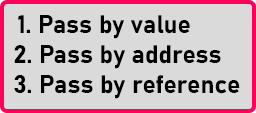These are the three methods supported in C++. If you know about C language that there were only two methods pass by value and pass by reference. But in C++ there are 3 methods: pass by value, pass by address and pass by reference. We can also call them as call by value, call by address, and call by reference.

So, by taking one single example, we will explain to you the difference between these 3 methods how they work. And once you know about these methods then you can use them based on your requirement, whichever is suitable you can use that.

##### Parameter Passing Technique – Pass by Value in C++:

Let us understand Parameter Passing Technique – Pass by Value in C++ with examples. Please have a look at the below code.

void swap(int a, int b){
int temp;
temp = a;
a = b;
b = temp;
}
int main(){
int x = 10, y = 20;
swap(x, y);
cout << x << ” ” << y;
}

let us understand the code first then we look at all the parameters passing methods one by one. Here inside the main function, we have two variables ‘x’ and ‘y’ and they are having some values ‘10’ and ‘20’. On the next line, we are calling the ‘swap’ function.

The ‘swap’ function will interchange the values of ‘x’ and ‘y’. So ‘y’ will become ‘10’ and ‘x’ will become ‘20’. After the call part, we are printing the values of ‘x’ and ‘y’. ‘swap’ function is taking 2 ‘int’ type parameters. So, values of ‘x’ and ‘y’ will be passed. This parameter passing method is call by value. Now we know well that the memory is divided into 2 sections. One is a code section in which the machine code of this program is residing as shown in the below image.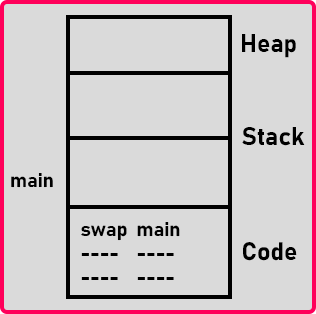Now let us start the execution. The program will start from the main function, this is the entry part of a program. So, the very first line says that we need two variables ‘x’ and ‘y’ to store some numbers. So, inside the stack, a block of memory is allocated for ‘x’ having the value ‘10’ and ‘y’ having the value ‘20’. We call this an activation record as shown in the below image.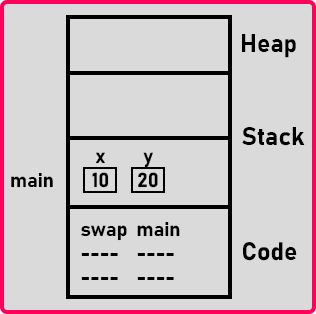Now next ‘swap’ function is called. This function has two parameters i.e. ‘a’ and ‘b’ and also inside that there is a variable ‘temp’, so a block of memory is allocated for the swap function as well in the stack memory. Now as this function is called, the value of ‘x’ is copied in ‘a’, and ‘y’ is copied in ‘b’. For a better understanding of the memory, please have a look at the below image.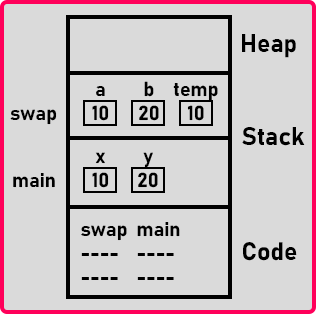Now let’s see what’s happening here. The value of a and b are interchange or ‘temp’ assign ‘a’ value. So ‘10’ will be copied in ‘temp’ then ‘a’ assign ‘b’ value so ‘b’ value is stored in ‘a’. Then ‘b’ assigned ‘temp’ so ‘b’ will contain the value of ‘temp’. Now at the last line or the moment when the function ends, we know well that once the function ends its activation record or the block of memory inside the stack will be deleted as shown in the below image.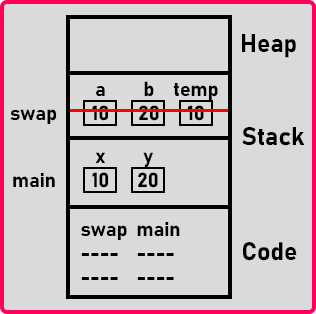Now the control comes back to the main function. It will print ‘x’ and ‘y’. The values of ‘x’ and ‘y’ are ‘10’ and ‘20’. They are not swapped. So, this is call by value method. In this method, the values of these ‘x’ and ‘y’ are copied in ‘a’ and ‘b’, and swapping of these variables is done but not with actual parameters. So that’s the point here in the call by value method. So ‘a’ and ‘b’ parameters we call them as actual parameters and ‘x’ and ‘y’ parameters we call them as formal parameters.

The value of the actual parameter is copied into the formal parameters. And if any changes are done inside the formal parameters will not change the actual parameters. So, the call-by-value mechanism cannot modify actual parameters.

##### When you use call by value mechanism?

If you want a function to just take the values and perform the operation and return the result then you use call by value. Call by value method is not suitable for the swap function. Now let us see the complete program.

##### Program to understand Pass by Value in C++
```#include <iostream>
using namespace std;
void Swap(int a, int b)
{
cout << "Before Swapping:" << endl;
cout << "a: " << a << " b: " << b << endl;
int temp;
temp = a;
a = b;
b = temp;
cout << "After Swapping:" << endl;
cout << "a: " << a << " b: " << b << endl;
}

int main ()
{
int x = 10, y = 20;
swap (x, y);
cout << "x: " << x << " y: " << y << endl;
}
```
###### Output: